Ph.D. in Economics, Associate Professor Kalashnikova T.V.

National Research Tomsk Polytechnic University, Russia

The synthesis of the demand-oriented pricing method with the parametric method

The purpose of this research is the development of models combining the demand-oriented pricing method with the parametric method.

First, the basic range of «profitable» price changes is needed to be calculated with the use of price elasticity coefficients. The establishment of the price in that range leads to the growth of the target figure (turnover or gross margin) relative to the level corresponding to the last price used in the analysis. Then the final price is determined with the help of parameters of the additive nature.

Legend: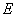– price elasticity coefficient,– consumer price,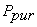– purchasing price.

The turnover of specific goods can be increased:

1)  if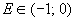, boosting the price by Y%,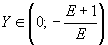;

2)if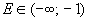, lowering the price by Y%,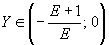;

3)if– the price is optimal.

The gross margin of specific goods can be increased:

1)  if, enhancing the price by Y%,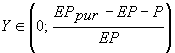;

2)  if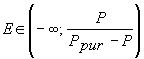, cutting the price by Y%,;

3)  if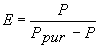– the price is optimal.

The selected parameters of points of sales:

1.   The number of competitors in a given radius of the shop (it is recommended to use a radius in the range of 500-1000 m)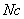.

In general, the more competitors, the lower the price will be established.

2.   The number of checks.

The more the quantity of checks, the higher the price will be fixed. Statistics of the number by quarters are used. It is necessary to determine the minimum and maximum values among all points of sales.

3. The average check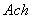.

Data of average checks by quarters are also used.

The same integer scale with an odd number of divisions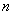is needed to be selected for the first three parameters. The minimum value of the scale is taken as the zero.

The scale for the parameter «number of competitors»:

«0» –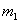and more competitors;

«1» –competitors;

«2» –competitors;

«n-2» –competitors;

«n-1» –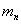competitors,

where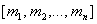– a set numbers of competitors.

The scale for the parameter «number of checks»:

«0» – quantity of checks for the last quarter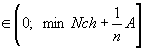;

«1» – quantity of checks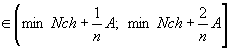;

«2» – quantity of checks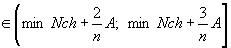;

«n-2» – quantity of checks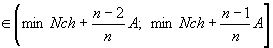;

«n-1» – quantity of checks,

where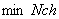– minimum number of checks for a quarter among all points of sales,– maximum number of checks for a quarter,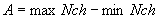.

The scale for the parameter «average check»:

«0» – value of the average check for the last quarter;

«1» – value of the average check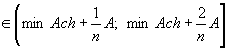;

«2» – value of the average check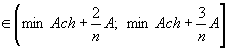;

«n-2» – value of the average check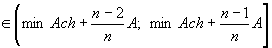;

«n-1» – value of the average check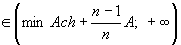,

где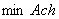– minimum value of the average check for the a quarter among all points of sales, \$;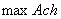– maximum value of the average check, \$;.

All three parameters are supposed to complement each other. So, the total parameter of a point of sales is defined as a sum of its individual parameters:,

where– parameter «number of competitors»,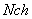– parameter « number of checks»,– parameter «average check».

If three integer scales have n divisions, and the minimum value is 0, the number of steps of the scale of the general parameter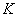is defined as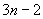.

The corridor of «profitable» price changes for a whole price group, found with the use of a price elasticity coefficient, should be divided into several equal ranges, contact with one of which depends on the value of the total parameter of the outlet. The quantity of bands is.

So as dependence functions of change of turnover and gross margin from price elasticity of demand have the parabolic character and the negative coefficient a, the optimal price lies in the middle of the price corridor found with the help of the elasticity.

The upper and lower limits of the range are determined depending on the total parameter of a point of sales: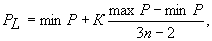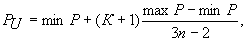where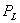– lower border of the range, \$;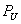– upper border of the range, \$;– the total parameter of a point of sales,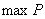– upper border of the corridor of «profitable» prices, \$;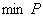– lower border of the corridor of «profitable» prices, \$.

·     If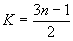(that is upon conditions of working points of sales, which are considered to be optimal) the optimal price of a point of sales coincides with the optimal price of the corresponding price group and lies in the middle of the corridor;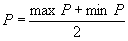;

·     if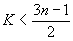optimal price – the upper limit of the corridor;

·     ifoptimal price – the lower limit of the corridor.

So, the pricing models, which allow determining a consumer price in the range of «profitable» prices taking into account individual characteristics of points of sales finally, have been developed.

References

1.   Martijn Brons, Peter Nijkamp, Eric Pels, Piet Rietveld. A meta-analysis of the price elasticity. A system of equations approach // Tinbergen Institute Discussion Paper. – 2006. – №106/3.

2.   Joseph G. Eisenhauer, Kristine E. Principe. Price knowledge and elasticity// Journal of Empirical Generalisations in Marketing Science. – 2009. – Vol 12, №2. – P. 24-35.

3.   Paul B. Ellickson, Sanjog Misra. Supermarket pricing strategies // Marketing Science. – 2008. – Vol 27, №5. – P. 811-828.